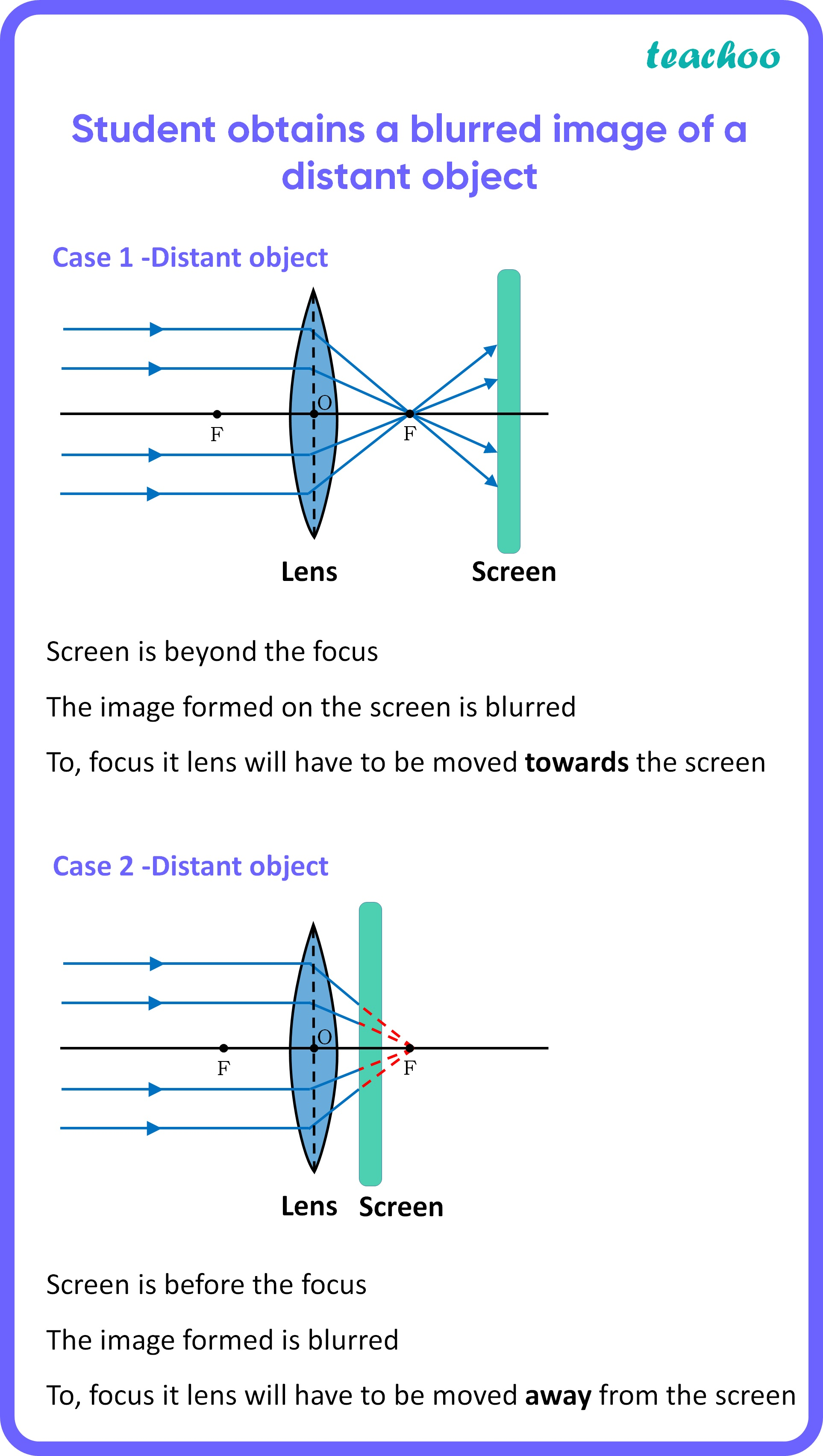MCQ from Past Year Papers

Class 10
Chapter 10 Class 10 - Light - Reflection and Refraction (Term 1)

## (d) either towards or away from the screen depending upon the position of the object

For a convex lens ,

• Since it is a distant object, it means object is at infinity
• Thus, image will be formed at focus
• Now, we don’t know where the screen is.
• The incident rays coming from the  distant object will be parallel to the principal axis   and as we know the rays parallel to the principal  axis, after refraction by convex lens, will pass   through the principal focus .

Hence, a distinct image will be obtained immediately when image distance (between screen and lens) is equal to focal length .Since we don’t know where the screen is, we could either move towards or away from the screen depending upon the position of the object.

So, the correct answer is (d)